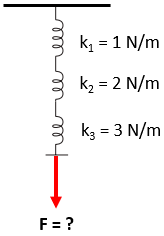## Springs in Series

Consider three springs in series are attached to a fixed point on a ceiling as shown in the figure. How much force is required to stretch the springs 5 m?Hint
The force in a spring is:
$$F_s=k\delta$$$where $$k$$ is the spring constant, and $$\delta$$ is the change in spring length from the un-deformed spring length. Hint 2 The spring constant for springs in series: $$\frac{1}{k_{eq}}=\sum_{i}\frac{1}{k_i}$$$
First, let’s determine the equivalent spring constant for several springs in series:
$$\frac{1}{k_{eq}}=\sum_{i}\frac{1}{k_i}$$$Since the problem statement has three springs in series: $$\frac{1}{k_{eq}}=\frac{1}{k_1}+\frac{1}{k_2}+\frac{1}{k_3}=\frac{1}{1N/m}+\frac{1}{2N/m}+\frac{1}{3N/m}$$$
$$\frac{1}{k_{eq}}=\frac{6}{6N/m}+\frac{3}{6N/m}+\frac{2}{6N/m}=\frac{11m}{6N}$$$$$\frac{1}{\frac{1}{k_{eq}}}=\frac{1}{\frac{11m}{6N}}$$$
$$k_{eq}=\frac{6}{11}\:N/m$$$A spring’s deflection and force are related by: $$F_s=k_{eq}\delta$$$
where $$F_s$$ is the forced applied to the spring, $$k_{eq}$$ is the equivalent spring constant, and $$\delta$$ is the change in spring length from the un-deformed spring length.
Therefore, the force required to stretch the springs 5 m is:
$$F=\frac{6N}{11m}\times 5m=2.7\:N$$\$
2.7 N